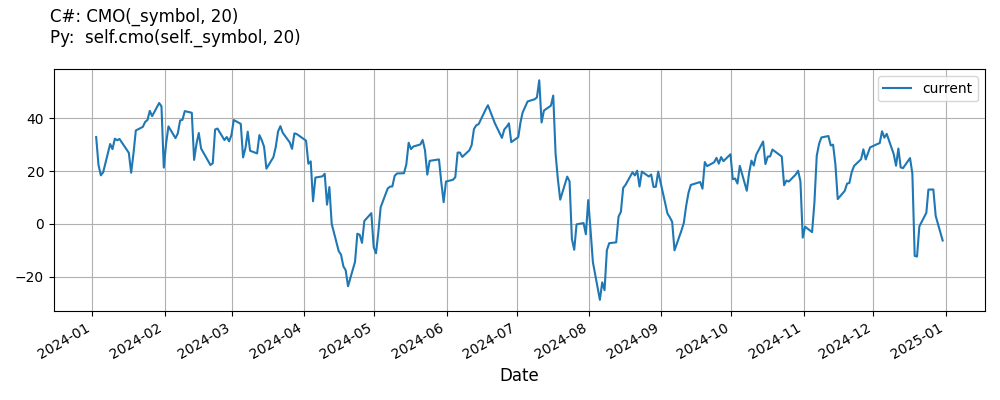# Supported Indicators

## Chande Momentum Oscillator

### Introduction

This indicator computes the Chande Momentum Oscillator (CMO). CMO calculation is mostly identical to RSI. The only difference is in the last step of calculation: RSI = gain / (gain+loss) CMO = (gain-loss) / (gain+loss)

To view the implementation of this indicator, see the LEAN GitHub repository.

### Using CMO Indicator

To create an automatic indicators for ChandeMomentumOscillator, call the CMO helper method from the QCAlgorithm class. The CMO method creates a ChandeMomentumOscillator object, hooks it up for automatic updates, and returns it so you can used it in your algorithm. In most cases, you should call the helper method in the Initialize method.

public class ChandeMomentumOscillatorAlgorithm : QCAlgorithm
{
private Symbol _symbol;
private ChandeMomentumOscillator _cmo;

public override void Initialize()
{
_cmo = CMO(_symbol, 20);
}

public override void OnData(Slice data)
{
{
// The current value of _cmo is represented by itself (_cmo)
// or _cmo.Current.Value
Plot("ChandeMomentumOscillator", "cmo", _cmo);

}
}
}
class ChandeMomentumOscillatorAlgorithm(QCAlgorithm):
def Initialize(self) -> None:
self.cmo = self.CMO(self.symbol, 20)

def OnData(self, slice: Slice) -> None:
# The current value of self.cmo is represented by self.cmo.Current.Value
self.Plot("ChandeMomentumOscillator", "cmo", self.cmo.Current.Value)



The following reference table describes the CMO method:

### CMO()1/1

            ChandeMomentumOscillator QuantConnect.Algorithm.QCAlgorithm.CMO (
Symbol                           symbol,
Int32                            period,
*Nullable<Resolution>      resolution,
*Func<IBaseData, Decimal>  selector
)


Creates a new ChandeMomentumOscillator indicator.

If you don't provide a resolution, it defaults to the security resolution. If you provide a resolution, it must be greater than or equal to the resolution of the security. For instance, if you subscribe to hourly data for a security, you should update its indicator with data that spans 1 hour or longer.

You can manually create a ChandeMomentumOscillator indicator, so it doesn't automatically update. Manual indicators let you update their values with any data you choose.

Updating your indicator manually enables you to control when the indicator is updated and what data you use to update it. To manually update the indicator, call the Update method with time/number pair, or an IndicatorDataPoint. The indicator will only be ready after you prime it with enough data.

public class ChandeMomentumOscillatorAlgorithm : QCAlgorithm
{
private Symbol _symbol;
private ChandeMomentumOscillator _cmo;

public override void Initialize()
{
_cmo = new ChandeMomentumOscillator(20);
}

public override void OnData(Slice data)
{
if (data.Bars.TryGeValue(_symbol, out var bar))
{
_cmo.Update(bar.EndTime, bar.Close);
}

{
// The current value of _cmo is represented by itself (_cmo)
// or _cmo.Current.Value
Plot("ChandeMomentumOscillator", "cmo", _cmo);

}
}
}
class ChandeMomentumOscillatorAlgorithm(QCAlgorithm):
def Initialize(self) -> None:
self.cmo = ChandeMomentumOscillator(20)

def OnData(self, slice: Slice) -> None:
bar = slice.Bars.get(self.symbol)
if bar:
self.cmo.Update(bar.EndTime, bar.Close)

# The current value of self.cmo is represented by self.cmo.Current.Value
self.Plot("ChandeMomentumOscillator", "cmo", self.cmo.Current.Value)



To register a manual indicator for automatic updates with the security data, call the RegisterIndicator method.

public class ChandeMomentumOscillatorAlgorithm : QCAlgorithm
{
private Symbol _symbol;
private ChandeMomentumOscillator _cmo;

public override void Initialize()
{
_cmo = new ChandeMomentumOscillator(20);
RegisterIndicator(_symbol, _cmo, Resolution.Daily);
}

public override void OnData(Slice data)
{
{
// The current value of _cmo is represented by itself (_cmo)
// or _cmo.Current.Value
Plot("ChandeMomentumOscillator", "cmo", _cmo);

}
}
}
class ChandeMomentumOscillatorAlgorithm(QCAlgorithm):
def Initialize(self) -> None:
self.cmo = ChandeMomentumOscillator(20)
self.RegisterIndicator(self.symbol, self.cmo, Resolution.Daily)

def OnData(self, slice: Slice) -> None:
# The current value of self.cmo is represented by self.cmo.Current.Value
self.Plot("ChandeMomentumOscillator", "cmo", self.cmo.Current.Value)



The following reference table describes the ChandeMomentumOscillator constructor:

### ChandeMomentumOscillator()1/2

            ChandeMomentumOscillator QuantConnect.Indicators.ChandeMomentumOscillator (
int  period
)


Initializes a new instance of the ChandeMomentumOscillator class using the specified period.

### ChandeMomentumOscillator()2/2

            ChandeMomentumOscillator QuantConnect.Indicators.ChandeMomentumOscillator (
string  name,
int     period
)


Initializes a new instance of the ChandeMomentumOscillator class using the specified name and period.

### Visualization

The following image shows plot values of selected properties of ChandeMomentumOscillator using the plotly library.You can also see our Videos. You can also get in touch with us via Discord.